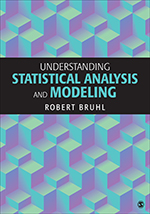# Understanding Statistical Analysis and Modeling

Understanding Statistical Analysis and Modeling is a text for graduate and advanced undergraduate students in the social, behavioral, or managerial sciences seeking to understand the logic of statistical analysis. Robert Bruhl covers all the basic methods of descriptive and inferential statistics in an accessible manner by way of asking and answering research questions. Concepts are discussed in the context of a specific research project and the book includes probability theory as the basis for understanding statistical inference. Instructions on using SPSS® are included so that readers focus on interpreting statistical analysis rather than calculations. Tables are used, rather than formulas, to describe the various calculations involved with statistical analysis and the exercises in the book are intended to encourage students to formulate and execute their own empirical investigations.

•••